# 3. Consider another Volterra integral equation (a) Solve the integral equation (4) by using the Laplace...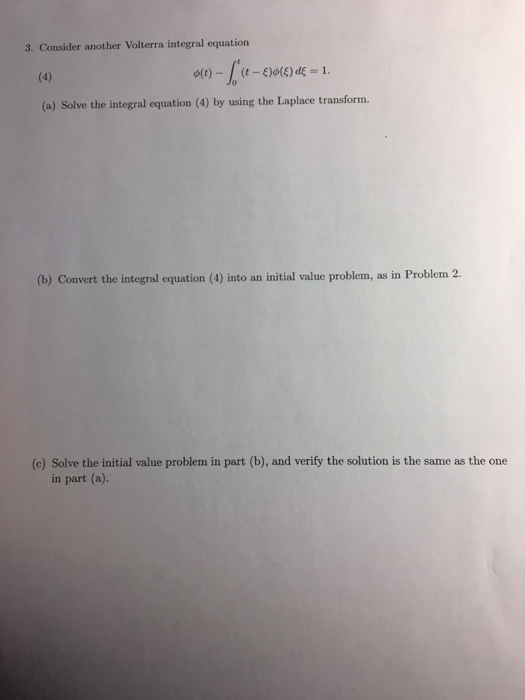3. Consider another Volterra integral equation (a) Solve the integral equation (4) by using the Laplace transform. (b) Convert the integral equation (4) into an initial value problem, as in Problem 2. (c) Solve the initial value problem in part (b), and verify the solution is the same as the one in part (a)

(a)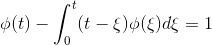Apply Laplace transform on both sides :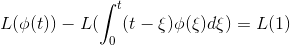Using the formula for Laplace transforms: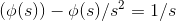=>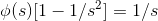=>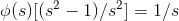=>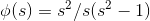=>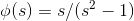=>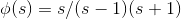{Factorizing denominator}

=>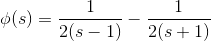{ Using partial fractions }

Taking inverse laplace transform: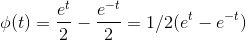(b) The given integral equation can also be written as :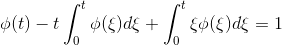=>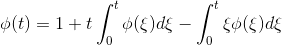Differentiating with respect to t :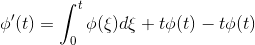=>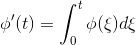Again differentiating with respect to t :

=>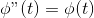=>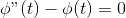The initial value problem is therefore: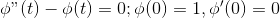(c) The characteristic equation is given by :

r2 -1 =0

=> r2 = 1 => r =-1, +1

The general solution is given by :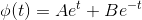=>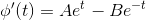Putting initial conditions: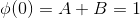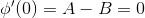Solving these equations , we get :

2A = 1 => A=1/2 and B = 1/2

Hence particular solution is given by :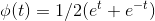This is same as we have got in part a)

#### Earn Coin

Coins can be redeemed for fabulous gifts.

Similar Homework Help Questions
• ### Use the Laplace transform to solve the Volterra integral equation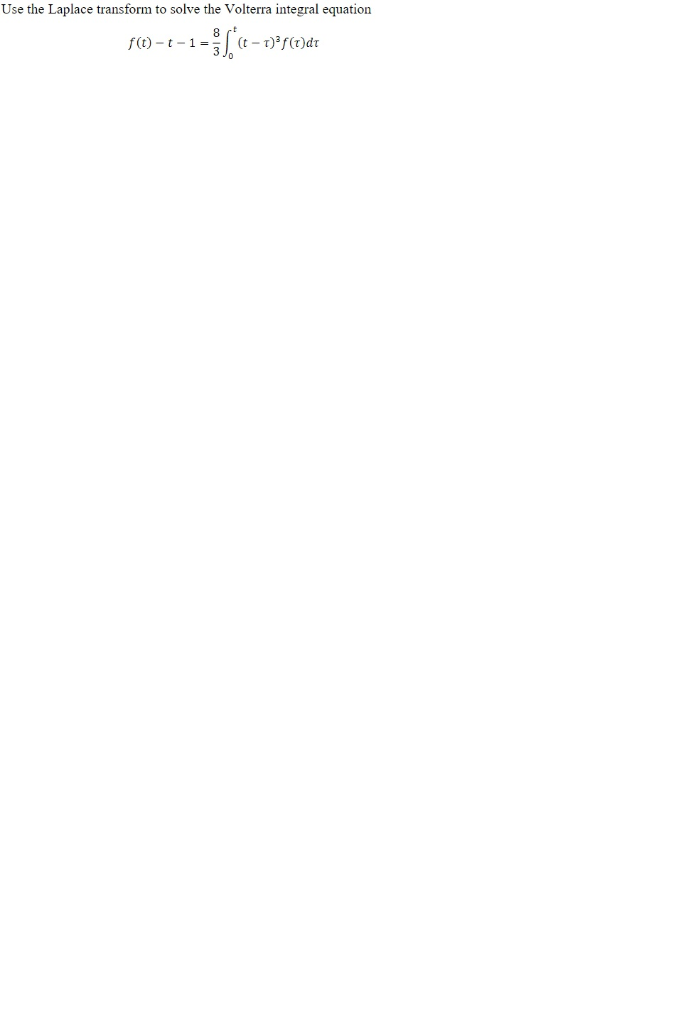Volterra Integral Equation Laplace Transform Use the Laplace transform to solve the Volterra integral equation Use the Laplace transform to solve the Volterra integral equation

• ### Problem 3. Consider the initial value problem w y sin() 0 Convert the system into a single 3rd order equation and solve resulting initial value problem via Laplace transform method. Express your answ...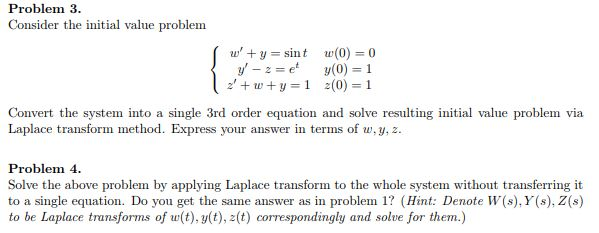Problem 3. Consider the initial value problem w y sin() 0 Convert the system into a single 3rd order equation and solve resulting initial value problem via Laplace transform method. Express your answer in terms of w,y, z. Problem 4 Solve the above problem by applying Laplace transform to the whole system without transferring it to a single equation. Do you get the same answer as in problem1? (Hint: Denote W(s), Y (s), Z(s) to be Laplace transforms of w(t),...

• ### 6. Solve an ODE Using Laplace Transforms: For this problem you are to use Laplace Transforms....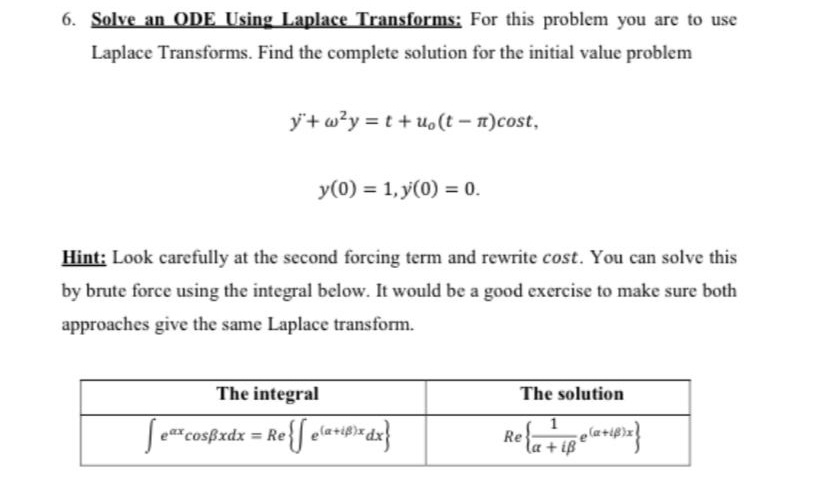6. Solve an ODE Using Laplace Transforms: For this problem you are to use Laplace Transforms. Find the complete solution for the initial value problem yº+w2y = t +u.(t - Ttcost, y(0) = 1, y(0) = 0. Hint: Look carefully at the second forcing term and rewrite cost. You can solve this by brute force using the integral below. It would be a good exercise to make sure both approaches give the same Laplace transform. The integral The solution ſeat...

• ### 4 points) In this exercise we will use the Laplace transform to solve the following initial value problem: y"-2y, + 17y-17, y(0)-0, y'(0)-1 (1) First, using Y for the Laplace transform of y(t...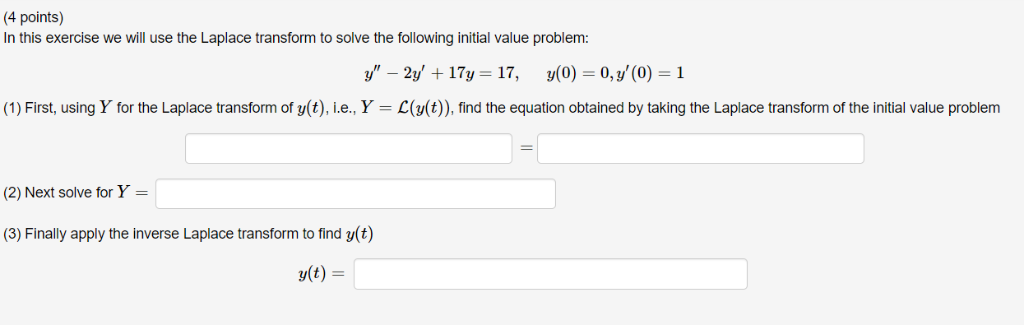4 points) In this exercise we will use the Laplace transform to solve the following initial value problem: y"-2y, + 17y-17, y(0)-0, y'(0)-1 (1) First, using Y for the Laplace transform of y(t), L.e., Y ()), find the equation obtained by taking the Laplace transform of the initial value problem (2) Next solve for Y- (3) Finally apply the inverse Laplace transform to find y(t) 4 points) In this exercise we will use the Laplace transform to solve the following...

• ### Consider the initial value problem y′+3y=10e^(7t)        y(0)=4. a. Take the Laplace transform of both sides...

Consider the initial value problem y′+3y=10e^(7t)        y(0)=4. a. Take the Laplace transform of both sides of the given differential equation to create the corresponding algebraic equation. Denote the Laplace transform of y(t) by Y(s). Do not move any terms from one side of the equation to the other (until you get to part (b) below). b. Solve your equation for Y(s). Y(s)=L[y(t)]= c. Take the inverse Laplace transform of both sides of the previous equation to solve for y(t)....

• ### Using the Laplace transform, solve the partial differential equation. Please with steps, thanks :) Problem 13: Solving...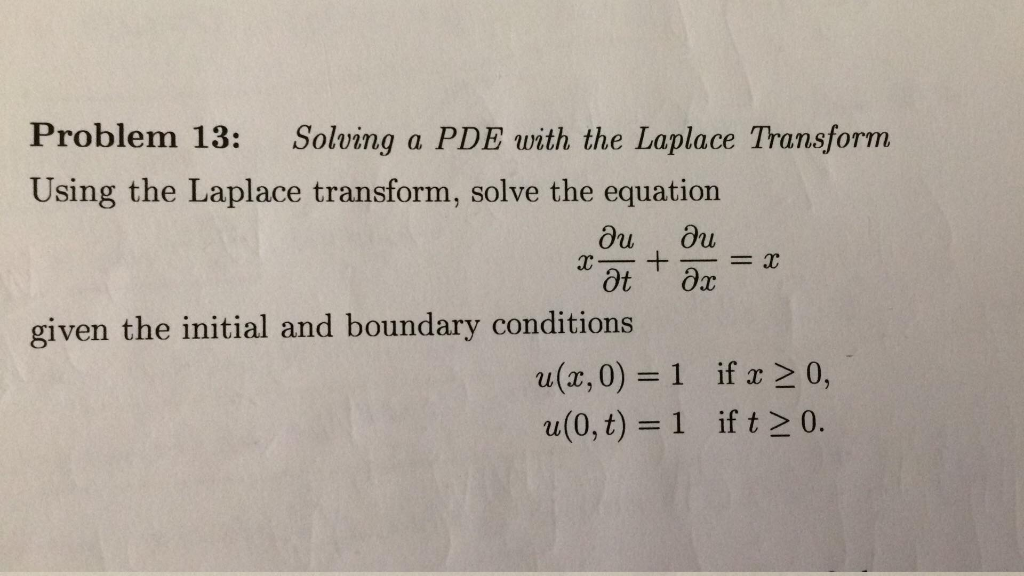Using the Laplace transform, solve the partial differential equation. Please with steps, thanks :) Problem 13: Solving a PDE with the Laplace Transform Using the Laplace transform, solve the equation 山 given the initial and boundary conditions a(x, 0)=1 ifx> 0, u(0, t) -1 if t 2 0. Problem 13: Solving a PDE with the Laplace Transform Using the Laplace transform, solve the equation 山 given the initial and boundary conditions a(x, 0)=1 ifx> 0, u(0, t) -1 if t...

• ### (t)= . Use the Laplace transform to solve the following initial value problem: 44" + 2y...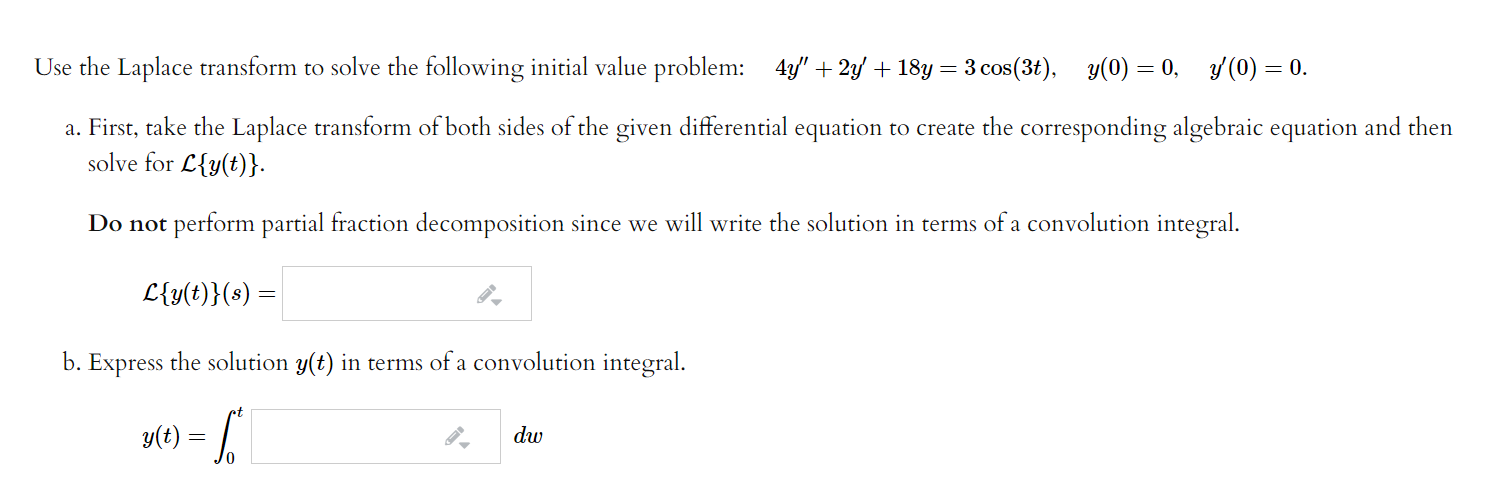(t)= . Use the Laplace transform to solve the following initial value problem: 44" + 2y + 18y = 3 cos(3+), y(0) = 0, y(0) = 0. a. First, take the Laplace transform of both sides of the given differential equation to create the corresponding algebraic equation and then solve for L{y(t)}. Do not perform partial fraction decomposition since we will write the solution in terms of a convolution integral. L{y(t)}(s) b. Express the solution y(t) in terms of a...

• ### (1 point) Consider the initial value problem 4y 8t, y(0) 4, y'(0) 3. f both sides of the given differential equatio...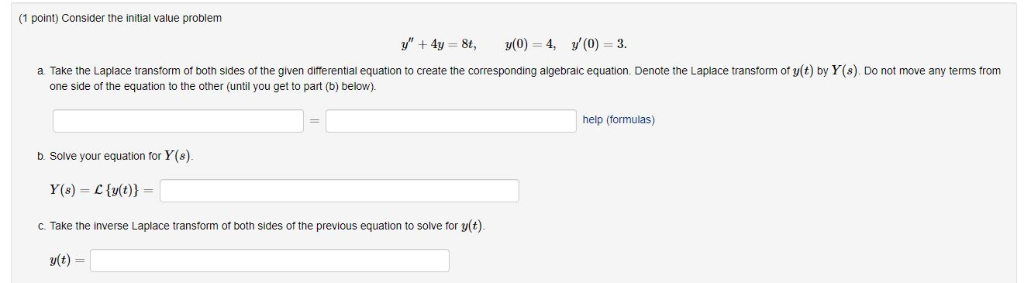(1 point) Consider the initial value problem 4y 8t, y(0) 4, y'(0) 3. f both sides of the given differential equation to create the corresponding algebraic equation. Denote the Laplace transform of y(t) by Y(s). Do not move any terms from othe other (until you get to part (b) below). Take the Laplace transform one side of the equation help (formulas) b. Solve your equation for Y(8) Y(s) C{y(t) = Take the inverse Laplace transform of both sides of the...

• ### Solve initial value problem using Laplace transform Problem 4 Solve the initial value problems given below...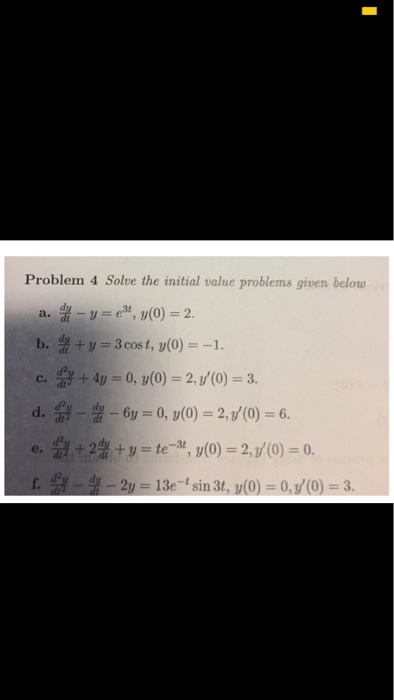Solve initial value problem using Laplace transform Problem 4 Solve the initial value problems given below --ез, y(0) 2. a. b. f ty 3 cos t, y(0)-